# Positive vector bundle

(diff) ← Older revision | Latest revision (diff) | Newer revision → (diff)

A generalization of the concept of a divisor of positive degree on a Riemann surface. A holomorphic vector bundleover a complex spaceis called positive (denoted by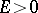) if onthere exists a Hermitian metricsuch that the function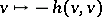onis strictly pseudo-convex outside the zero section. Ifis a manifold, the condition for positivity is expressed in terms of the curvature of the metric. That is, the curvature form of the metricin the bundlecorresponds to a Hermitian quadratic formonwith values in the bundle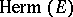of Hermitian endomorphisms of the bundle. The positivity condition is equivalent tobeing a positive-definite operator onfor anyand any non-zero.

Ifis a complex line bundle over a manifold, the condition for positivity is equivalent to that of positive definiteness of the matrix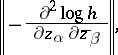where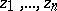are local coordinates inandis a function that defines the Hermitian metric for the local trivialization of the bundle. Ifis compact, then the complex line bundleoveris positive if and only if the Chern class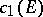contains a closed form of the type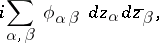where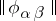is a positive-definite Hermitian matrix. In particular, ifis a Riemann surface, then the bundle overdefined by a divisor of degreeis positive if and only if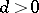. Ifis a bundle of rank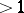over a manifoldof dimension, one can consider also the following narrower class of positive bundles: A bundleis called positive in the sense of Nakano if there exists ona Hermitian metricsuch that the Hermitian quadratic formon the bundle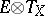as defined by the formula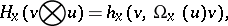where,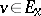and, is positive definite. Examples: the tangent bundle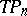of the projective space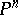is positive, but forit is not positive in the sense of Nakano; the complex line bundle overdefined by a hyperplane is positive.

Any quotient bundle of a positive vector bundle is positive. Ifandare positive bundles (in the sense of Nakano), then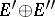and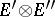are positive (in the sense of Nakano).

The concept of a positive bundle was introduced in connection with Kodaira's vanishing theorem (cf. Kodaira theorem) for complex line bundles, and it was then extended to any bundle. Somewhat later, in relation to the existence of an imbedding in a projective space, the concepts of weakly-positive and weakly-negative bundles were introduced.

A holomorphic vector bundleover a compact complex spaceis called weakly negative if its zero section has a strictly pseudo-convex neighbourhood in, i.e. if it is an exceptional analytic set. A bundleis called weakly positive if the dual bundle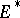is weakly negative. Ifis a Riemann surface, the concepts of a weakly-positive bundle and a positive bundle coincide . In the general case, positivity implies weak positivity; no examples are known at present (1983) of weakly positive but non-positive bundles.

Weak positivity of a bundle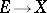is equivalent to each of the following properties: For any coherent analytic sheaf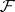onthere exists an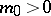such that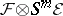for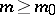is generated by global sections; for any analytic sheafonthere exists an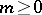such that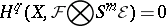for all, . By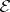is meant the sheaf of germs of holomorphic sections of the bundle. Weakly-positive bundles are therefore analogous to the ample sheafs (cf. Ample sheaf) from algebraic geometry and are sometimes called ample analytic bundles. A weakly-positive bundle over a spacenaturally defines an imbedding ofinto a Grassmann manifold and thus into a projective space.

The concepts of a positive, a negative, a weakly-positive, and a weakly-negative bundle are naturally extended also to the case of a linear space over a complex space(see Vector bundle, analytic).